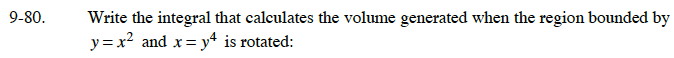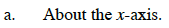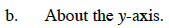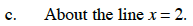### Home > CALC > Chapter 9 > Lesson 9.3.1 > Problem9-80

9-80.Sketch the region and determine the points of intersection.The outside radius (R) is the curve x = y4. The inside radius (r) is the curve y = x2. This will be a "dx" integral, so make sure your radii expressions are in terms of x.Identify which curve will be the outside radius and which curve will be the inside radius. This will be a "dy" integral, so make sure your radii expressions are in terms of y.Since x = 2 is a vertical line, this will be similar to part (b). This time the outside radius will be (2 – R) and the inside radius will be (2 – r).